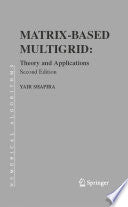# Matrix-based Multigrid: Theory And Applications (numerical Methods And Algorithms)

Free shipping (Guaranteed delivery 3-5 business days for In-Stock products)

## ISBN details

• ISBN 10: 0387497641
• ISBN 13: 9780387497648

## Overview

Matrix-Based Multigrid introduces and analyzes the multigrid approach for the numerical solution of large sparse linear systems arising from the discretization of elliptic partial differential equations. Special attention is given to the powerful matrix-based-multigrid approach, which is particularly useful for problems with variable coefficients and nonsymmetric and indefinite problems. This book can be used as a textbook in courses in numerical analysis, numerical linear algebra, and numerical PDEs at the advanced undergraduate and graduate levels in computer science, math, and applied math departments. The theory is written in simple algebraic terms and therefore requires preliminary knowledge only in basic linear algebra and calculus.

## Other Details

• Publisher: Springer
• Language: Eng English
• Format: print
• Edition: 2
• Dimensions: xxiii, 318 pages : illustrations ; 25 cm.
• Pages: 318
• Date Published: 2008
• Authors: Yair Shapira